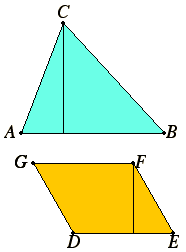# Definition 4

The height of any figure is the perpendicular drawn from the vertex to the base.

## Guide

 Evidently, what is meant by “vertex” is the highest point in the figure, or a highest point when many points are equally high. But to define “height” in terms of “highest point” would be a circular definition. Indeed, this definition only suggests what “height” might mean without defining it at all. Still, by the way the term is used in the Elements, we can determine its meaning. The only planar figures where heights are used in the Elements are triangles and parallelograms. If the figure is a triangle, and one side has been declared the base, then the height is the expected line, the line drawn from the opposite vertex perpendicular to the base. If the figure is a parallelogram, and one side has been declared the base, then the height may be taken to be a perpendicular from either of the two vertices not on the base. In the later books on solid geometry, other figures also can have bases and heights such as parallelepipeds, pyramids, prisms, cones, and cylinders.Different sides of a figure may be selected as the base depending on the application. In proposition XI.39 there are two triangular prisms. A triangle is chosen taken to be the base of one, while the base of the other is a parallelogram. The height of the first is a perpendicular drawn between two triangular opposite faces, but the height of the other is a perpendicular drawn between the parallelogram taken as the base and the opposite parallelogram.